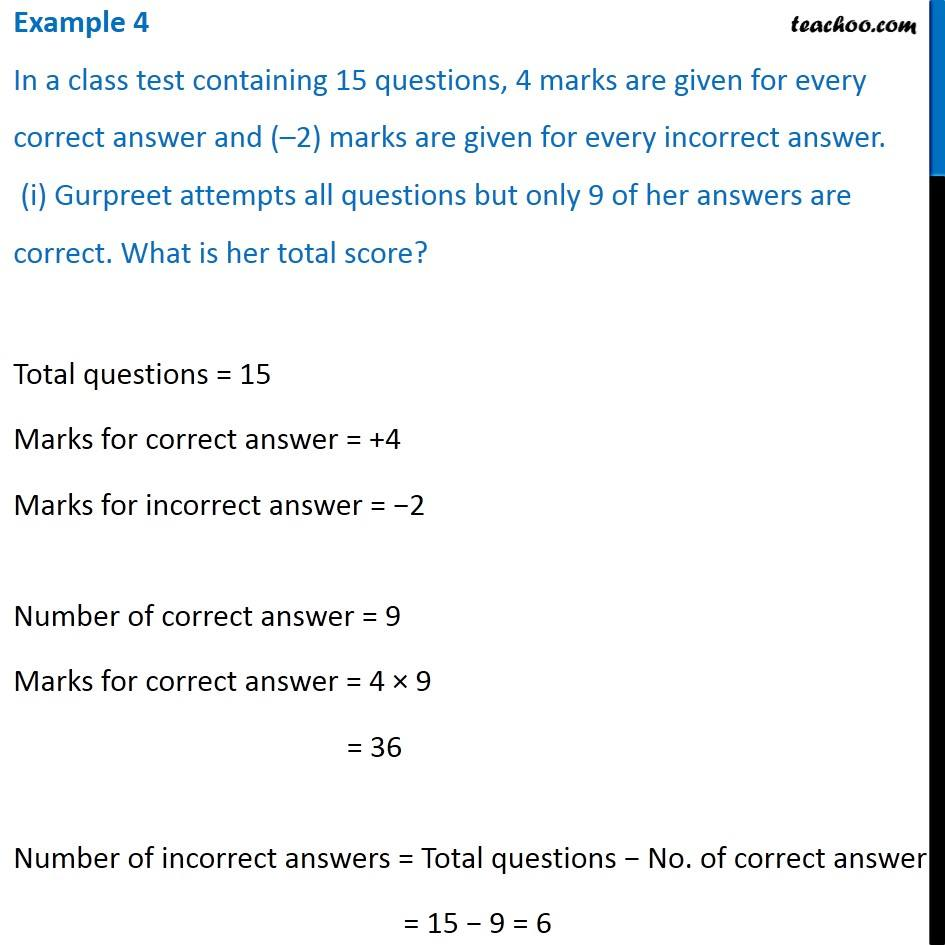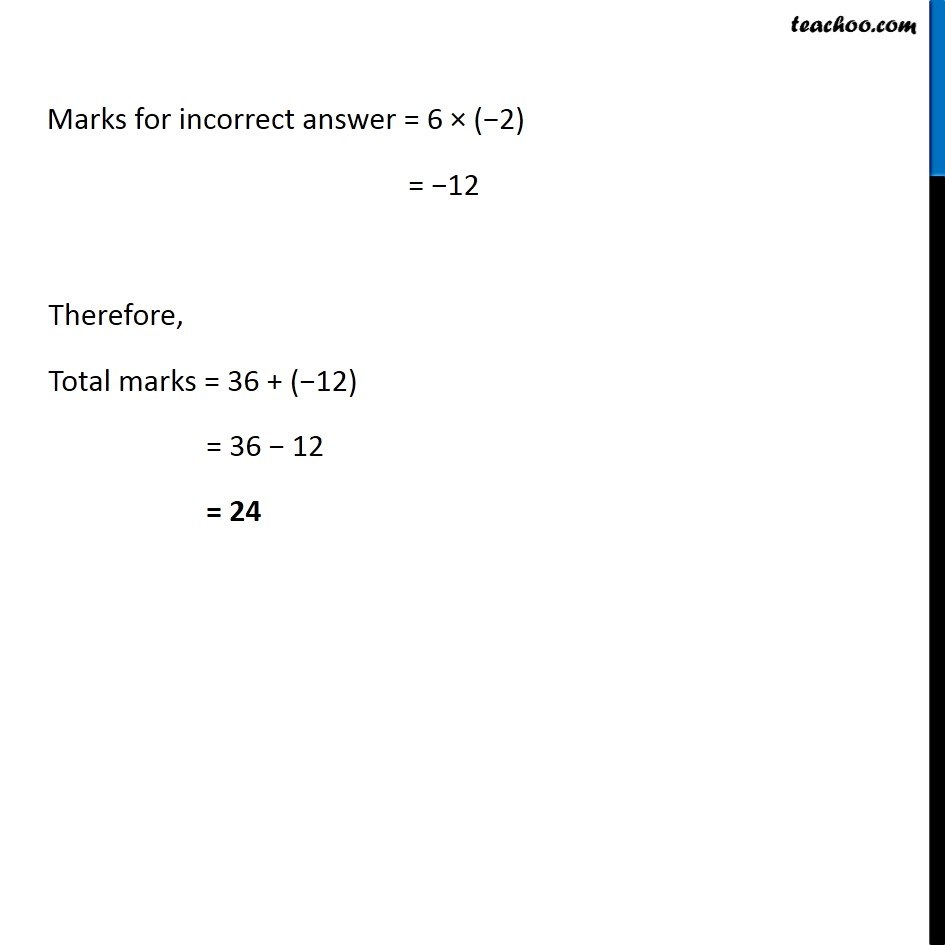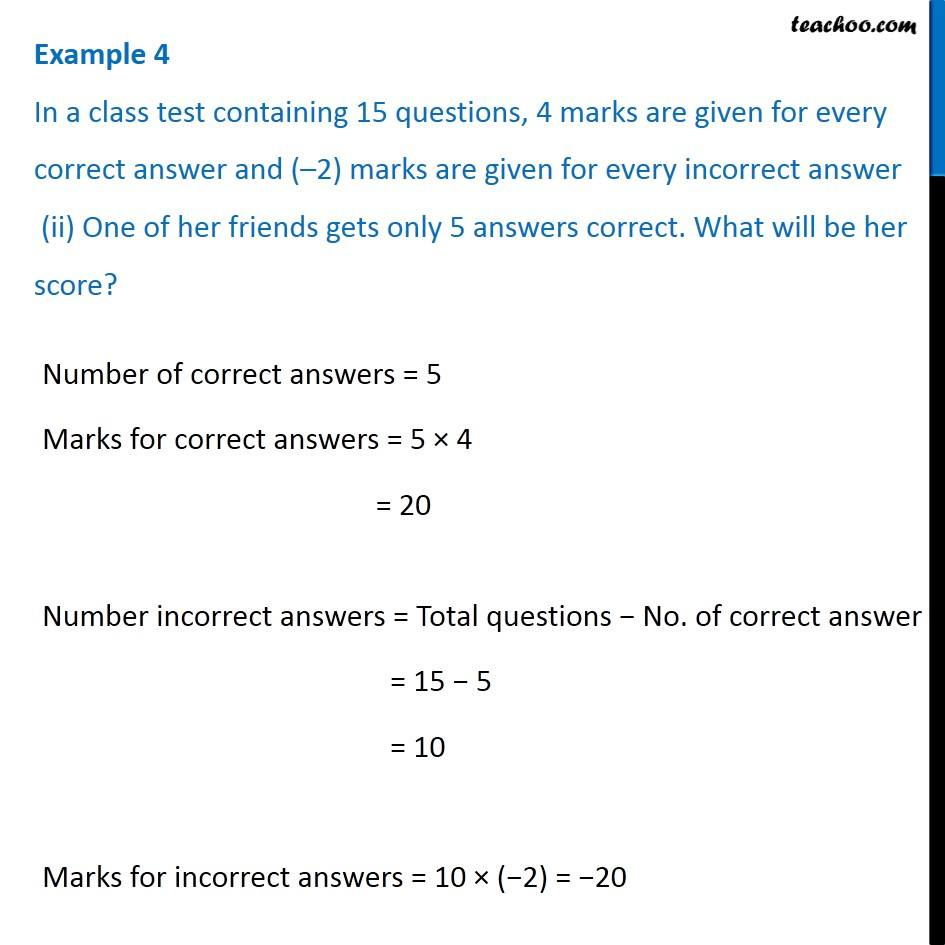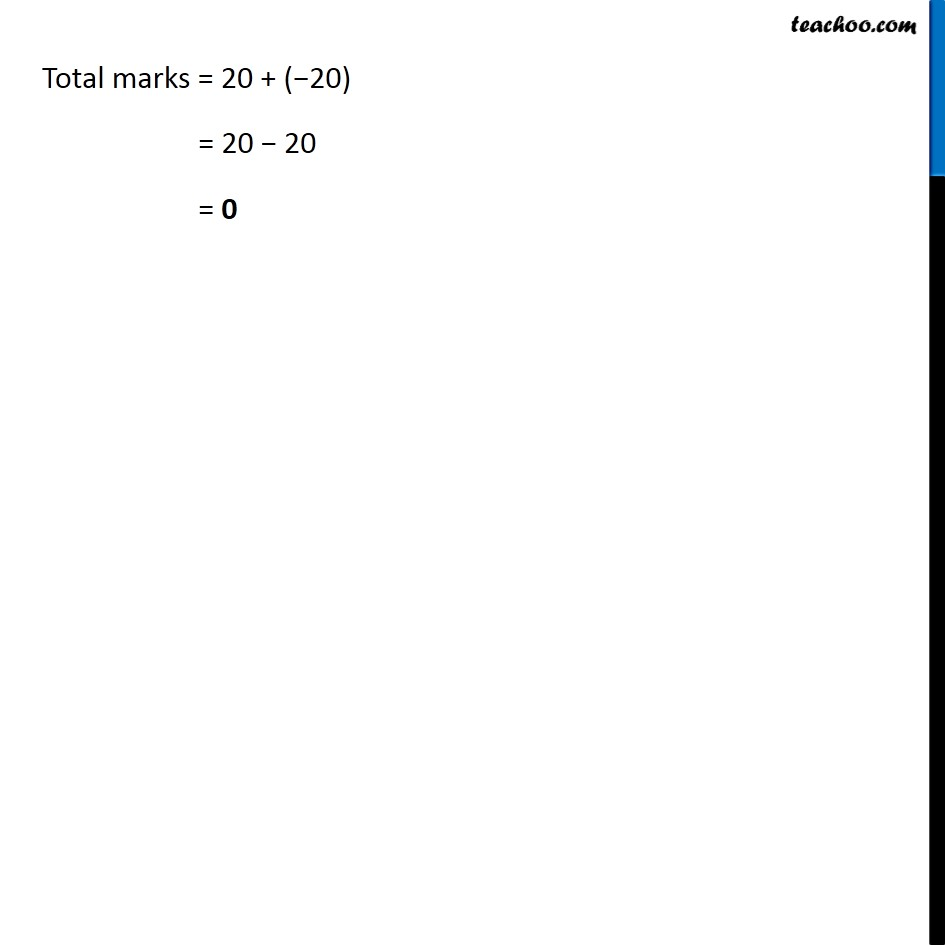1. Chapter 1 Class 7 Integers
2. Serial order wise
3. Examples

Transcript

Example 4 In a class test containing 15 questions, 4 marks are given for every correct answer and (–2) marks are given for every incorrect answer. (i) Gurpreet attempts all questions but only 9 of her answers are correct. What is her total score? Total questions = 15 Marks for correct answer = +4 Marks for incorrect answer = −2 Number of correct answer = 9 Marks for correct answer = 4 × 9 = 36 Number of incorrect answers = Total questions − No. of correct answer = 15 − 9 = 6 Marks for incorrect answer = 6 × (−2) = −12 Therefore, Total marks = 36 + (−12) = 36 − 12 = 24 Example 4 In a class test containing 15 questions, 4 marks are given for every correct answer and (–2) marks are given for every incorrect answer (ii) One of her friends gets only 5 answers correct. What will be her score? Number of correct answers = 5 Marks for correct answers = 5 × 4 = 20 Number incorrect answers = Total questions − No. of correct answer = 15 − 5 = 10 Marks for incorrect answers = 10 × (−2) = −20 Total marks = 20 + (−20) = 20 − 20 = 0

Examples